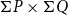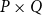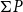Hostname: page-component-758b78586c-ddb2s Total loading time: 0 Render date: 2023-11-28T16:54:39.790Z Has data issue: false Feature Flags: { "corePageComponentGetUserInfoFromSharedSession": true, "coreDisableEcommerce": false, "useRatesEcommerce": true } hasContentIssue false

## Abstract

The study of the sobriety of Scott spaces has got a relatively long history in domain theory. Lawson and Hoffmann independently proved that the Scott space of every continuous directed complete poset (usually called domain) is sober. Johnstone constructed the first directed complete poset whose Scott space is non-sober. Soon after, Isbell gave a complete lattice with a non-sober Scott space. Based on Isbell’s example, Xu, Xi, and Zhao showed that there is even a complete Heyting algebra whose Scott space is non-sober. Achim Jung then asked whether every countable complete lattice has a sober Scott space. The main aim of this paper is to answer Jung’s problem by constructing a countable complete lattice whose Scott space is non-sober. This lattice is then modified to obtain a countable distributive complete lattice with a non-sober Scott space. In addition, we prove that the topology of the product space$\Sigma P\times \Sigma Q$ coincides with the Scott topology of the product poset$P\times Q$ if the set Id(P) and Id(Q) of all incremental ideals of posets P and Q are both countable. Based on this, it is deduced that a directed complete poset P has a sober Scott space, if Id(P) is countable and$\Sigma P$ is coherent and well filtered. In particular, every complete lattice L with Id(L) countable has a sober Scott space.

## Keywords

Type
Paper
Information
Mathematical Structures in Computer Science , October 2023 , pp. 809 - 831

## Access options

Get access to the full version of this content by using one of the access options below. (Log in options will check for institutional or personal access. Content may require purchase if you do not have access.)

## References

Goubault-Larrecq, J. (2013). Non-Hausdorff Topology and Domain Theory , New Mathematical Monographs, vol. 22, Cambridge, Cambridge University Press.Google Scholar
Gierz, G., Hofmann, K. H., Keimel, K., Lawson, J. D., Mislove, M. and Scott, D. S. (2003). Continuous Lattices and Domains , Encyclopedia of Mathematics and its Applications, vol. 93, Cambridge, Cambridge University Press.Google Scholar
Hochster, M. (1969). Prime ideal structure in commutative rings. Transactions of the American Mathematical Society 142 4360.CrossRefGoogle Scholar
Hoffmann, R.-E. (1981). Continuous posets, prime spectra of a completely distributive complete lattice, and Hausdorff compactifications. In: Continuous Lattices, Springer LNNM, vol. 871, 159–208.CrossRefGoogle Scholar
Isbell, J. (1982). Completion of a construction of Johnstone. Proceedings of the American Mathematical Society 85 333334.CrossRefGoogle Scholar
Jia, X., Jung, A. and Li, Q. (2016). A note on coherence of dcpos. Topology and Its Applications 209 235238.CrossRefGoogle Scholar
Jia, X. (2018). Meet-Continuity and Locally Compact Sober Dcpos. Phd thesis, University of Birmingham.Google Scholar
Johnstone, P. (1981). Scott is not always sober. In: Continuous Lattices, Lecture Notes in Mathematics, vol. 871, Springer-Verlag, 282–283.CrossRefGoogle Scholar
Jung, A. (2018). Four dcpos, a theorem, and an open problem, an invited talk in Hunan University, Hunan Province, China, 1 December 2018.Google Scholar
Lawson, J. (1979). The duality of continuous posets, Houston Journal of Mathematics 5 357386.Google Scholar
Xi, X. and Lawson, J. D. (2017) On well-filtered spaces and ordered sets. Topology and Its Applications 228 139144.CrossRefGoogle Scholar
Xu, X., Xi, X. and Zhao, D. (2021). A complete Heyting algebra whose Scott space is non-sober. Fundamenta Mathematicae 252 315323.CrossRefGoogle Scholar
Xu, X. and Zhao, D. (2020). Some open problems on well-filtered spaces and sober spaces. Topology and Its Applications 301 107540.CrossRefGoogle Scholar